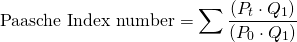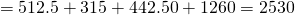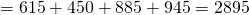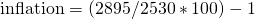# Paasche Index

The Paasche index (PI) is an index calculation method that is typically used to determine the rate of inflation. Thus, the PI is a consumer price index formula (CPI). It is not used as often as the Laspeyres index, even though it has one clear advantage compared to the Laspeyres index. The Paasche index was introduced by Hermann Paasche.

On this page, we present how to calculate the Paasche index, discuss a simple Paasche index example, and finally discuss the main advantage of the index. At the bottom of this page, we include a Paasche Index calculator using an Excel spreadsheet.

## Paasche quantity index formula

Paasche’s index number formula is the following:where Pt is the prices today, P0 is the prices in the previous period, and Q1 is the basket of goods today. For those who know the Laspeyres index, this formula will look very familiar. There is only one big difference though. Instead of using the quantities of the goods of last year, we use the current quantities. Other than that, the calculation is the same as that for the Laspeyres index.

On the page on the Laspeyres  index, we discuss the advantages and disadvantages of Laspeyres and Paasche price index. Here, we focus on the biggest advantage of the Paasche index. In particular, since the Paasche index uses current quantities of goods (i.e. current consumption), the Paasche takes into consideration the fact that consumers may be substitution between goods.

At the same time, there is also an important disadvantage to the use of the Paasche index. Just as the Laspeyres index will overestimate the inflation rate, the index can be expected to underestimate inflation. As consumers substitute to cheaper goods, those cheaper goods will get a higher weight than would otherwise be the case.

## Paasche price index example

Finally, let us consider a simple example: suppose we have 4 goods

• Oranges
• tram tickets
• milk
• necklaces

Suppose we have a basket of goods, then how do we calculate the Paasche index number? Suppose we buy 205 oranges, 45 tram tickets, 295 gallons of milk, and a 105 necklaces today. The prices for these goods last year were $2.5,$7.00, $1.50,$12.00, respectively. Today, the goods cost $3.00,$10.00, $3, and$9.00.

Applying the formula, we get the index for last yearThe index for this year isInflation equalsor 14.43 %

## Summary

We discussed the Paasche index, a commonly used CPI formula. It shows how to calculate a price index using current quantities of goods. At the bottom of this page, we include the consumer price index calculator based on the PI.

### Paasche index calculator

Want to have an implementation in Excel? Download the Excel file: Paasche Index